Reach Us+44-1522-440391
A Miscellaneous Note on the Equivalence of Two Poisson Likeliho ods | OMICS International
Journal of Biometrics & Biostatistics

Like us on:

All submissions of the EM system will be redirected to Online Manuscript Submission System. Authors are requested to submit articles directly to Online Manuscript Submission System of respective journal.

# A Miscellaneous Note on the Equivalence of Two Poisson Likeliho ods

Bohning D1* and Viwatwongkasem C2

1Southampton Statistical Sciences Research Institute, University of Southampton, Southampton SO17 1BJ, UK

2Department of Biostatistics, Faculty of Public Health Mahidol University, Bangkok, Thailand

*Corresponding Author:
Bohning D
Southampton Statistical Sciences Research Institute, University of Southampton
Southampton SO17 1BJ, UK
Tel: 00 44 (0)78 7951 9129
Fax: 00 44 (0) 23 8059 3216
E-mail: [email protected]

Received date: February 05, 2015; Accepted date: June 04, 2015; Published date: June 11, 2015

Citation: Bohning D, Viwatwongkasem C (2015) A Miscellaneous Note on the Equivalence of Two Poisson Likelihoods. J Biomet Biostat 6: 231. doi: 10.4172/2155-6180.1000231

Copyright: © 2015 Bohning D, et al. This is an open-access article distributed under the terms of the Creative Commons Attribution License, which permits unrestricted use, distribution, and reproduction in any medium, provided the original author and source are are credited.

Visit for more related articles at Journal of Biometrics & Biostatistics

#### Abstract

This note shows that the concept of an offset, frequently introduced in Poisson regression models to cope with ratetype data, can be simply treated with a regular Poisson regression model. Hence Poisson regression models requiring an offset can be fitted with ordinary Poisson regression models. Some illustrations are provided and it is discussed how this result came about.

#### Keywords

Poisson likelihood; Offset; Log-link

#### Introduction

The paper at hand has anecdotal character and tells a story what can happen in the life of a university professor. An exam question was posted involving a Poisson regression model with an offset term. Most students found the solution that was also provided by the professor as the official solution (to the exam office), except one student who ignored the offset term and treated it as failures. The solution was marked initially as false as one would evidently not work along this pathway. However, as was correctly claimed by the student, the solution he/she provided was entirely identical with the solutions by his/her colleagues that were marked as correct. The professor sat down and worked out what was going on, and after two days, not without interruptions of the typical university daily life, it was found that also the unorthodox solution has a right to live as both likelihoods could be shown to identical. The purpose of this note is to share this experience.

We consider the data constellation as provided in Table. This is done entirely for illustrative reasons and does not provide any limitations on the generality of the major finding. Here a count (number of events, say) Yi is considered for a binary exposure Ei and observation-time at risk Ti where i indicates the unit and goes from 1 to n. The person-time Ti is considered non-random

Whereas Yi is a discrete random variable. This situation is commonly denoted as a rate-type problem with rate

λi=E(Yi )/Ti.

This rate leads to a natural modelling as follows

log E(Yi)=log Ti+log λi        (1)

The term log Ti is a covariate with known coefficient and called offset whereas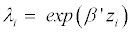contains the linear predictor with covariate vector zi for the ith unit. For the situation of Table 1, with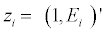the model would simply be

log E(Yi )=log Ti01 Ei,

uniti Yi Ei Ti
1 2 1 3
2 4 1 5
3 6 1 7
4 3 0 4
5 4 0 5
6 1 0 3

Table 1: Hypothetical data for six units arising from a cohort study with response count Y, binary exposure E and person-time T.

where β1 is the log-risk ratio, usually the parameter of interest . If one assumes that Yi is Poisson with mean E(Yi) given by (1), then the associated log-likelihood is given by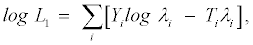(2)

where we have ignored parameter independent terms. Note that the full log-likelihood (including parameter independent parts) is log L1+Yi log Ti-log(Yi!). Many packages exist that can fit offset models such as (1). For the data of Table 1 we use STATA (2013) to yield the output given in Figure 1 .

#### A Second Problem

Consider a second problem where we observe, for each unit i, a binary variate Xij where j=1,…, Ti . In other words, Xij=1 if the event of interest occurs and zero otherwise, for j=1, …, Ti. Let, for unit i,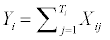denote the count of positives and Ti-Yi are the number of zeros. Let pij denote the probability for an event in the ith subject at the jth occasion. Then the clustered likelihood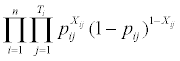arises, assuming independence over subjects and occasions. This simplifies further if the event probability is constant over occasion: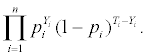Let us make the unconventional assumption, purely for the mathematical convenience of showing the equivalence, that Xij follows a Poisson distribution with mean λi. In other words, we assume that

log E(Xij)=log λi             (3)

for i =, …, n and j=1, … , Ti , and Xij∼P o(λi). In particular, this implies P(Xij=1)=pi=exp(-λii and P (Xij=0)=1-pi=exp(-λi). Then, the following associated likelihood (Corresponding also to the full likelihood) occurs: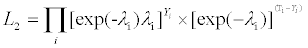(4)

Model (3) can be fitted by regressing a 2n vector of n 1s and n 0s, (1,…, 1, 0, … , 0)i , on the 2n vector(s) of associated covariates, for example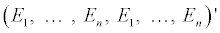in the situation of Table 2, using as frequency weight vector the 2n-vector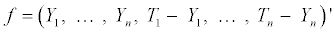. This can be done easily in STATA and yields the output in Figure 2. Note that the model (3) does not involve any offset. It is clear from Figures 1 and 2 that the results of the analysis for model (1) and (3) are identical. We show in the following that this is not accidental (as it might have only occurred in this special data set) but is far more general in nature.

unit i Ei Xij fi
1 1 1 2
2 1 1 4
3 1 1 6
4 0 1 3
5 0 1 4
6 0 1 1
1 1 0 1
2 1 0 1
3 1 0 1
4 0 0 1
5 0 0 1
6 0 0 2

Table 2: The data of Table 1 reorganized: for the first set of n observations: let for each unit i fi=Yi=#{Xij=1} denote the count of 1s in the binary set Xi1, · · · ,XiTi and for the second set of n observations: let for each unit i fi=Ti-Yi=#{Xij=0} denote the number of 0s.

#### Results

We have the following result:

Theorem 1:

log L1=log L2.

Proof: We start with the clustered log-likelihood (4) and show that it is identical to the log-likelihood (2):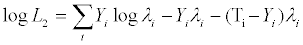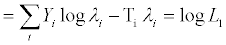which ends the proof.

Note that this result, although mathematical almost trivial, is quite general and does not depend in any way on the form of linear predictor. Note that the full (including all terms) log-likelihoods are not identical as also Figures 1 and 2 indicate. Typically, the full loglikelihood corresponding to model (1) will be larger, assuming Yi ≤ Ti, since

Yi log Ti≥ Yi log Yi≥ log(Yi!)

for i=1, … , n.

• We would like to mention that the result does not require Ti to be an integer. The log-likelihood remains well-defined even if Ti is of real value as it might occur with rate data or otherwise. All that is required is that Ti ≥ Yi which is only a question of scaling for T.

• The result does not require a special form of linear predictor. However, it is does not generalized beyond the log-link, typical for Poisson regression or log-linear modelling.

#### Discussion

The result might have more curiosity than impact, but is interesting in it. It means, for example, that we can fit offset models without paying attention to the special offset variate in (1), but simply use the conventional model

log E(Xij)=log λi,                            (5)

Where, for each unit i, Xij=1 exactly Yi -times and Ti-Yi times otherwise. The question arises how this result can be used. We see at least two applications:

• It can be used to fit Poisson-offset models in packages that do not provide the offset-option.

• It may be used to check the computational correctness of the offset option if the latter is available.

However, the most important take-home message might be that one needs to be careful when to decide about the correctness of a solution provided by an unorthodox thinking student. Finally, we wish to mention that the student received the full mark despite remaining doubts that he/she fully understands the depth of the equivalence.

#### References

Select your language of interest to view the total content in your interested language

### Article Usage

• Total views: 12023
• [From(publication date):
June-2015 - Sep 17, 2019]
• Breakdown by view type
• HTML page views : 8235

## Post your commentCan't read the image? click here to refresh
###### Peer Reviewed Journals

Make the best use of Scientific Research and information from our 700 + peer reviewed, Open Access Journals

International Conferences 2019-20

Meet Inspiring Speakers and Experts at our 3000+ Global Annual Meetings

Top